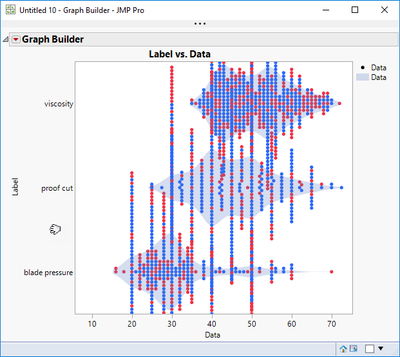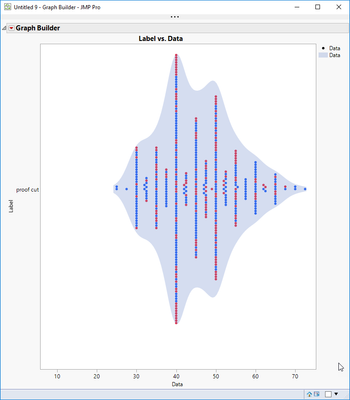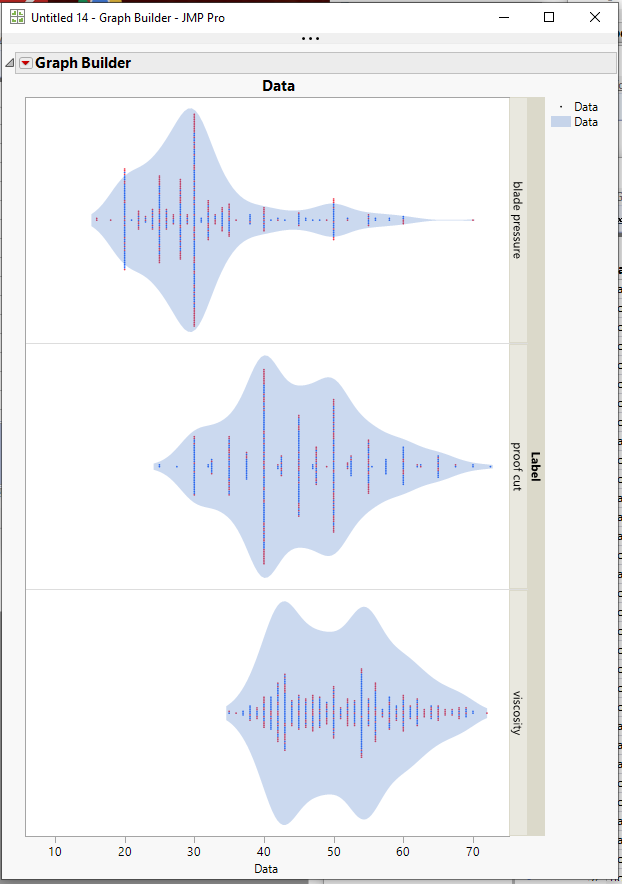Choose Language Hide Translation Bar
Highlighted

## Plotting packed distribution with equal width (Jitter = 0)

When using violin plots (density), there is an option to have equal widths per variable.

However, I am unable to plot the dot distribution with the same shape as it does not respect the width of each variable.``````// Start of script;
Names Default To Here( 1 );
Clear Log();

dt = Open( "\$SAMPLE_DATA/Bands Data.JMP" );

Data Table( "Bands Data" ) << Stack(
columns( :proof cut, :viscosity, :blade pressure ),
Source Label Column( "Label" ),
Stacked Data Column( "Data" ),
Drop All Other Columns( 1 )
);

Graph Builder(
Size( 534, 456 ),
Show Control Panel( 0 ),
Variables( X( :Data ), Y( :Label ) ),
Elements(
Points( X, Y, Legend( 5 ), Jitter( "Packed" ), Jitter Limit( 0 ) ),
Contour( X, Y, Legend( 6 ), Violin Scaling( "Equal Width" ) )
)
)``````

I would like to know how to achieve something like this but for a number of variables.2 REPLIES 2
Highlighted

## Re: Plotting packed distribution with equal width (Jitter = 0)

I like the concept.  You should add it to the JMP Wish List

The closest I could come to the chart you want is by removing the Label variable from the Y axis, and making it a Y Grouping variable.  Then I changed the marker size to a dot.  Finally I changed the aspect ratio of the chart by stretching it making the display taller.Jim
Highlighted

## Re: Plotting packed distribution with equal width (Jitter = 0)

Thanks. So, as it seems this is not possible for JMP 15:

Here is the proposal for those that are interested: Plotting packed distribution with equal width and no jitter

Article Labels